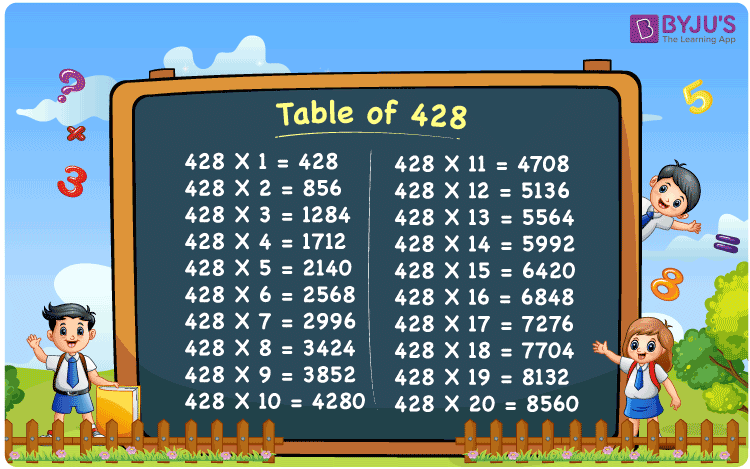Checkout JEE MAINS 2022 Question Paper Analysis : Checkout JEE MAINS 2022 Question Paper Analysis :

# Table of 428

The table of 428 is a multiplication table that consists of 428 and natural numbers multiplied together. Learning the 428 times table will help you do your arithmetic computations faster. In this article, you can find a 428 times chart as well as a tabular version of the multiplication table of 428. You can access a PDF of the table of 428 by clicking the link below.

## Table of 428 Chart## What is the 428 Times Table?

Up to the first ten times, the table of 428 using the repeated addition and multiplication methods is presented below.

 428×1 = 428 428 428×2 = 856 428 + 428 = 856 428×3 = 1284 428 + 428 + 428 = 1284 428×4 = 1712 428 + 428 + 428 + 428 = 1712 428×5 = 2140 428 + 428 + 428 + 428 + 428 = 2140 428×6 = 2568 428 + 428 + 428 + 428 + 428 + 428 = 2568 428×7 = 2996 428 + 428 + 428 + 428 + 428 + 428 + 428 = 2996 428×8 = 3424 428 + 428 + 428 + 428 + 428 + 428 + 428 + 428 = 3424 428×9 = 3852 428 + 428 + 428 + 428 + 428 + 428 + 428 + 428 + 428 = 3852 428×10 = 4280 428 + 428 + 428 + 428 + 428 + 428 + 428 + 428 + 428 + 428 = 4280

## Multiplication Table of 428

The multiplication table of 428 for the first 20 natural numbers is given here.

 428 × 1 = 428 428 × 2 = 856 428 × 3 = 1284 428 × 4 = 1712 428 × 5 = 2140 428 × 6 = 2568 428 × 7 = 2996 428 × 8 = 3424 428 × 9 = 3852 428 × 10 = 4280 428 × 11 = 4708 428 × 12 = 5136 428 × 13 = 5564 428 × 14 = 5992 428 × 15 = 6420 428 × 16 = 6848 428 × 17 = 7276 428 × 18 = 7704 428 × 19 = 8132 428 × 20 = 8560

## Solved Example on the Table of 428

Example:

What is 28 more than the 10th multiple of 428?

Solution:

Using the multiplication table of 428, the 10th multiple of 428 is 4280.

I.e., 428 × 10 = 4280.

Hence, 28 more than the 10th multiple of 428 = 28 + 4280 = 4308.

## Frequently Asked Questions on the Table of 428

### What is the table of 428?

428×1 = 428, 428×2 = 856, 428×3 = 1284, 428×4 = 1712, 428×5 = 2140, 428×6 = 2568, 428×7 = 2996, 428×8 = 3424, 428×9 = 3852, 428×10 = 4280.

### How many times does 428 equal 4708?

11 times 428 equals 4708.

### What is 428 times 20?

428 times 20 is 8560. I.e., 428 × 20 = 8560.